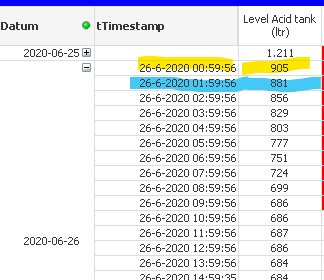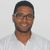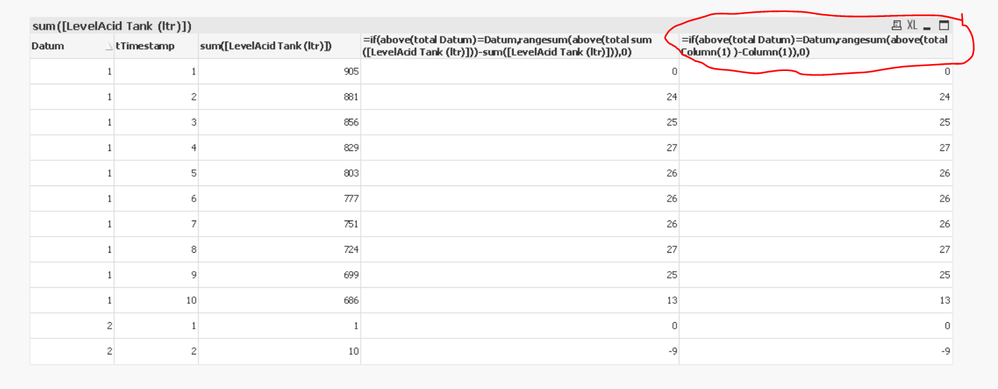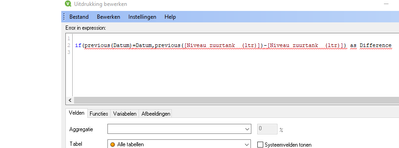Announcements
You can succeed best and quickest by helping others to succeed. Join the conversation.
cancel
Showing results for
Did you mean:Contributor III

## How to calculate difference of the value between two records in a table

I have several tanks with level measurment. Beside of showing the level in the tank I also want to show the amount of liquid used between two timestamps.

How can I calculate this usage?

With regards

Jan Droog1 Solution

Accepted SolutionsMVP

Yes!

as you can replace the measure calculated by Column(1) in your case.

look at this example, I replaced the measure (in your case AVG.....)with Column(1)

``=if(above(total Datum)=Datum,rangesum(above(total Column(1) )-Column(1)),0)``Regards,
Taoufiq ZARRA

"Please LIKE posts and "Accept as Solution" if the provided solution is helpful "

(you can mark up to 3 "solutions") 😉
6 RepliesMVP

Hi,

One solution :

``if(previous(Datum)=Datum,previous([LevelAcid Tank (ltr)])-[LevelAcid Tank (ltr)]) as Difference``

for example for this sample :

``````load *,if(previous(Datum)=Datum,previous([LevelAcid Tank (ltr)])-[LevelAcid Tank (ltr)]) as Difference  inLine [
Datum,tTimestamp,LevelAcid Tank (ltr)
1,1,905
1,2,881
1,3,856
1,4,829
1,5,803
1,6,777
1,7,751
1,8,724
1,9,699
1,10,686
2,1,1
2,2,10
];``````

the output :Regards,
Taoufiq ZARRA

"Please LIKE posts and "Accept as Solution" if the provided solution is helpful "

(you can mark up to 3 "solutions") 😉Contributor III
Author

Got the example working, but I need this calculation in the Expression box.

It that also possible?MVP

One solution

Dimension :

Datum,tTimestamp,LevelAcid Tank (ltr)

Expression :

``=if(above(total Datum)=Datum,rangesum(above(total sum([LevelAcid Tank (ltr)]))-sum([LevelAcid Tank (ltr)])),0)``

output :Regards,
Taoufiq ZARRA

"Please LIKE posts and "Accept as Solution" if the provided solution is helpful "

(you can mark up to 3 "solutions") 😉Contributor III
Author

Got this one running.

Is it also possible when LevelAcid-Tank is a calculated value?

With regards, JanMVP

Yes!

as you can replace the measure calculated by Column(1) in your case.

look at this example, I replaced the measure (in your case AVG.....)with Column(1)

``=if(above(total Datum)=Datum,rangesum(above(total Column(1) )-Column(1)),0)``Regards,
Taoufiq ZARRA

"Please LIKE posts and "Accept as Solution" if the provided solution is helpful "

(you can mark up to 3 "solutions") 😉Contributor III
Author

YES!!

That's exactly what i needed.  😀

Thank you very much for helping me out.

Jan DroogCommunity Browser